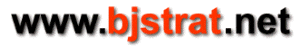# Computing the probability of a running count for a counting system

## Blackjack Counting System

Blackjack is dealt from 1 or more standard playing card decks. Generally the number of decks is 1, 2, 4, 6, or 8 and the undealt cards are usually referred to as a shoe. A freshly shuffled number of decks is called a full shoe. In blackjack the ranks of the cards are A (ace,) 2, 3, 4, 5, 6, 7, 8, 9, T (ten.) The T rank consists of 10, jack, queen, king and each of these cards is equivalent to the other. A counting system divides these ranks into 2 or more groups and assigns a numerical value called a tag to each card in the group.

## Running Count

The running count (RC) of a counting system is defined as sum of (number of cards present in each group) times (tag of that group). The initial running count (IRC) is the running count of a freshly shuffled shoe. The probability of a running count occurrence at any point in the deal of a shoe can be computed given the following information:
1. number of decks
2. number of cards remaining to be dealt
3. running count
4. optionally number of each rank known to be specifically removed (due to inclusion in player and/or dealer hand)

## HiLo Counting System

HiLo is the most popular blackjack counting system and is generally a standard due to its simplicity while at the same time having a reasonable degree of effectiveness. HiLo consists of 3 count groups
{2,3,4,5,6} tag = -1
{7,8,9} tag = 0
{T,A} tag = +1
It will be shown how to compute the probability of a HiLo RC of 0 with 26 cards remaining dealt from a single deck shoe. The same principles apply to any counting system, number of decks, cards remaining, and RC.

## Example: Computing the probability of a HiLo RC = 0 dealt from a single deck

```General mathematics involved:
let n = a given number of cards, let x = a given number of cards where x <= n
1. number of ways a given number of cards can be dealt considering order = n*(n-1)*...*1
n*(n-1)*...*1 is denoted n!
example: 5! = 5*4*3*2*1 = 120
2. number of ways x cards chosen from n cards can be dealt where order doesn't matter
denote above as C(n,x)
C(n,x) = n*(n-1)*...*(n-x+1) / (n-x)!
example C(6,3) = 6*5*4/3! = 120/6 = 20

Mathematics independent of counting system:
let d = number of decks, let maxCards = maximum number of undealt cards = 52*d
let cardsRemaining = number of cards remaining to be dealt
for single deck d = 1, maxCards = 52, cardsRemaining is variable from 52 to 1

Mathematics specific to HiLo counting system:
let l = number of (2,3,4,5,6) as a group, let max_l = max number of (2,3,4,5,6) = 20*d
let m = number of (7,8,9) as a group, let max_m = max number of (7,8,9) = 12*d
let h = number of (T,A) as a group, let max_h = max number of (T,A) = 20*d
for single deck max_l = 20, max_m = 12, max_h = 20
define a HiLo subset as a group of cards with l low cards, m medium cards, and h high cards
denote a HiLo subset as s(l,m,h) (number of cards in subset = l+m+h)

Computing the probability of a subset:
given a HiLo subset s(l,m,h)
let pSubset = probability of s
let numCards = l+m+h
pSubset = C(max_l,l)*C(max_m,m)*C(max_h,h) / C(maxCards,numCards)

Computing the probability of a given RC for a given number of undealt cards:
1. compile a list of all subsets with both the given RC and the given number of undealt cards
2. compute pSubset for each subset in the list and sum them

Specific example - HiLo RC=0 with 26 cards remaining to be dealt from single deck:
list of subsets: s1(7,12,7), s2(8,10,8), s3(9,8,9), s4(10,6,10),
s5(11,4,11), s6(12,2,12), s7(13,0,13)

pSubset1 = C(20,7)*C(12,12)*C(20,7) / C(52,26) = 1.2117616101733490e-005
pSubset2 = C(20,8)*C(12,10)*C(20,8) / C(52,26) = 0.0021118732812302398
pSubset3 = C(20,9)*C(12,8)*C(20,9) / C(52,26) = 0.028158310416403209
pSubset4 = C(20,10)*C(12,6)*C(20,10) / C(52,26) = 0.063600237127182685
pSubset5 = C(20,11)*C(12,4)*C(20,11) / C(52,26) = 0.028158310416403189
pSubset6 = C(20,12)*C(12,2)*C(20,12) / C(52,26) = 0.0021118732812302398
pSubset7 = C(20,13)*C(20,13) / C(52,26) = 1.2117616101733488e-005

prob(RC=0) = pSubset1 + pSubset2 + pSubset3 + pSubset4 + pSubset5 + pSubset6 + pSubset7
prob(RC=0) = 0.12416483975465302

Allow for specific removals:
If for any reason you want to allow for additional cards known to be removed such as cards in
player's hand or dealer up card you will need to adjust one or more of the parameters
max_l, max_m, max_h (re HiLo) and also maxCards depending upon what is specifically removed.
(Also the list of possible subsets may change.)

Example: adjust probabilities for a hand of A-2 versus dealer up card of 4 (single deck)
max_l = 20 - 2 = 18 (2 and 4 are in low card group; total low cards is 18 instead of 20)
max_h = 20 - 1 = 19 (A is in high card group; total high cards is 19 instead of 20)
maxCards = 52 - 3 = 49 (3 cards removed from full shoe; total number of cards is 49 instead of 52)

Computation is the same as above except the altered parameters are used.
```

## Other counting systems/number of decks/cards remaining/running count

Above methodology can be extrapolated to other counting systems/number of decks/cards remaining/running count.